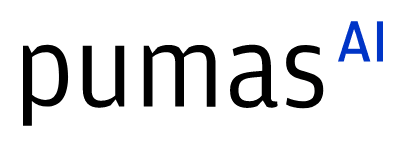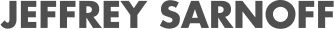# FdeSolver.jl: Solving fractional differential equations

07/27/2022, 2:50 PM3:00 PM UTC
Blue

#### Abstract:

FdeSolver Julia package solves fractional differential equations in the sense of Caputo, suitable for nonlinear and stiff ordinary differential systems. It has been used for describing memory effects in microbial community dynamics and complex systems. With some practical examples, I will present why (and how) developing such a computational package in open source programming is important (and useful).

#### Description:

Differential equations with fractional operators describe many real-world phenomena more accurately than integer order calculus. Fractional calculus has been recognized as a powerful method for understanding the memory and nonlocal correlation of dynamic processes, phenomena, or structures. However, the Julia programming language has missed a package for solving differential equations of fractional order in an accurate, reliable, and efficient way. Hence, we devise the FdeSolver Julia package specifically for solving two general classes of fractional-order problems: fractional differential equations (FDEs) and multi-order systems of FDEs. We implement the explicit and implicit predictor-corrector algorithms with sufficient convergence and accuracy of the solution of the nonlinear systems, including the fast Fourier transform technique that gives us high computation speed and efficient treatment of the persistent memory term. The following document provides some overall information for using the package: https://juliaturkudatascience.github.io/FdeSolver.jl/stable/readme## Media partners## Community partners